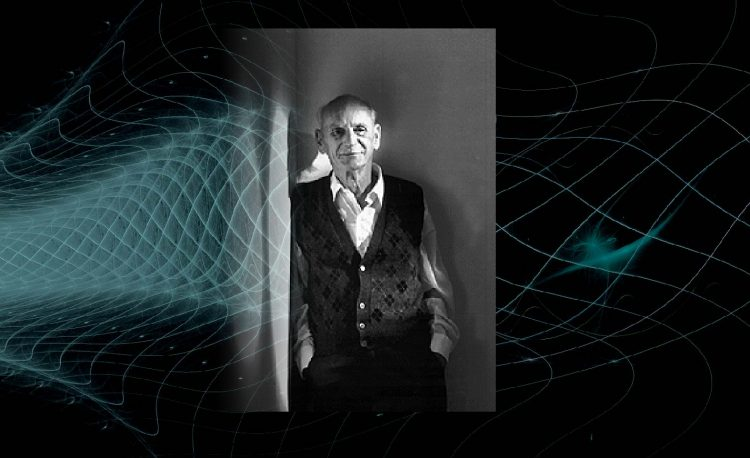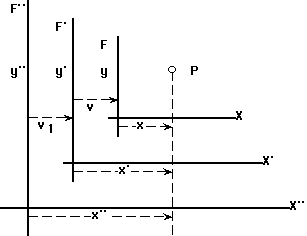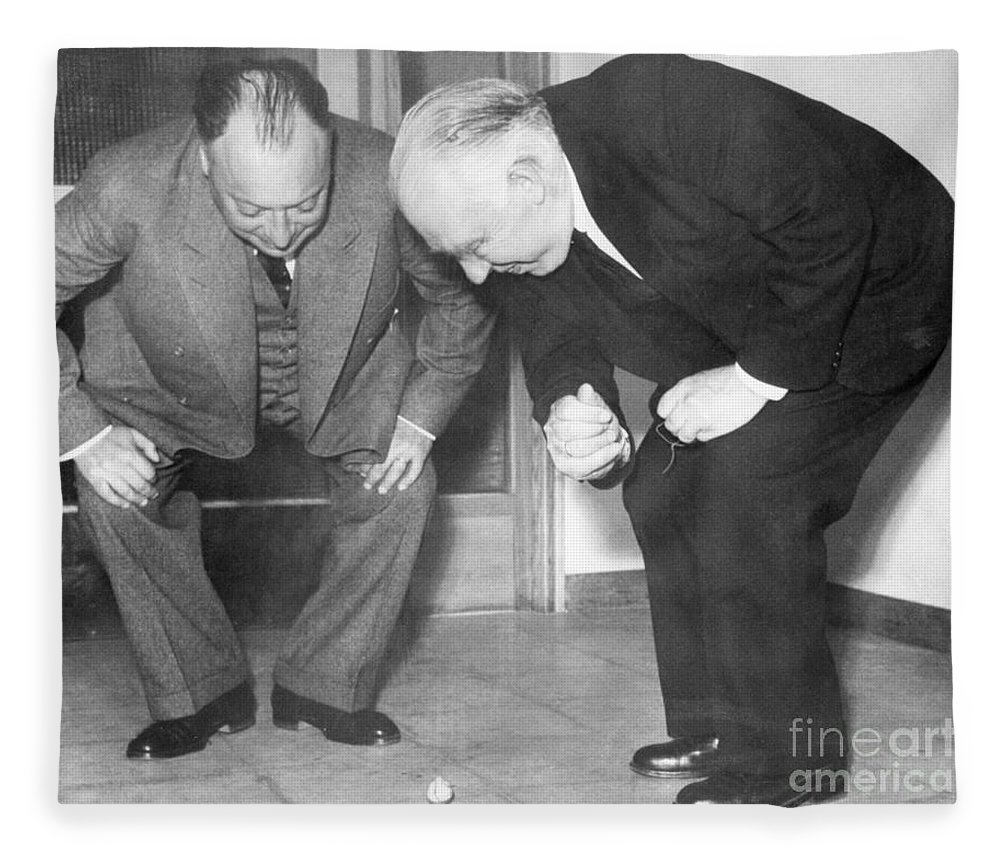# Applying the Wrong Equations and Einstein

In 1940, in a physics class in Argentina, a simple question from a physics student to his professor became a moment of “eureka” that was to take this physics student on a journey of decades challenging Albert Einstein himself. Einstein was 60 years old at the time and already hailed as a genius but this student was to change everything with a simple insight that seemed very innocent at the time.

The question was simple: why were physicists applying a kinetic energy equation to decay or radioactivity? After all, there is no outside energy in the case of radioactivity. A radioactive material decays without any external energy – it is all internal. Yet, kinetic energy is an equation that describes an external force being applied to a system.

Young Carezani’s question confounded the professor because the entire argument of the lesson was to apply Einstein’s kinetic energy equations or “relativistic kinematic equations” to cases of decay or radioactivity. Carezani’s question didn’t even involved Einstein and relativity. It was a simple case of the very definition of kinetic energy and decay.

Not being satisfied with the way the discussion was going, Carezani went to work on his own. After an intense 4 years of work from the age of 20 to 24, he emerged with new equations to replace Einstein’s special relativity equations. And to arrive at those new sets of equations which took relativity back to the world of Newton’s classical mechanics, a second “eureka” moment occurred.

### Extra Frames

In the derivations for Einstein’s special relativity equations, young Carezani found out that both mathematically, and physically, there was no need for two or more frames of reference.In deriving Einstein’s equations for special relativity, at least two frames are need yet Carezani found that more than one frame was redundant.

What this meant was that when measuring the movement of any object in the universe, the observer of the movement should measure this movement directly from their own frame of reference, not through a “second” or “third” reference frame (reference frames being coordinate systems).

Einstein’s special relativity is based on the assumption that there exists “inertial frames” or reference for all moving objects and that in these inertial frames, all the same laws of physics apply. Carezani, during his 4 years, came to realize that in fact, setting up coordinate systems on every moving object was mathematically redudent and recreated an artificial separation of the velocity of an object that was then set equal to the velocity of the second coordinate system, and that lead to an error in one of the famous derivations for Einstein’s equations in describing the well known Lorentz equations.

In layman terms: measuring the velocity of an object through more than one coordinate system “broke” special relativity’s derivation of Lorentz’s famous equations.

Carezani’s “eureka” moment was that there were no such things as inertial frames or more than one three dimensional space. There is only one three dimensional space where everything in the universe exists and moving objects can only correctly be measured “directly” between a moving object and the observer. All we can truly do is to move our one coordinate system to some place in the universe and measure from there.

### No Neutrino

Carezani first recognized that kinetic energy equations or any type – even Einstein’s – cannot be applied to decay cases which gave off energy without any outside interference or energy. When stopped applying the special relativity kinetic energy equations and replaced them with Carezani’s new “autodynamic” equations, Pauli’s invented Neutrino – the bad boy of particle physics – was no longer needed.Wolfgang Pauli with Neils Bohr – Carezani was to unravel the mystery of the neutrino and use that to dervice Bohr’s atomic equations.

These new equations also simplified particle physics in that it no longer needed special relativity and could rely on Newtonian equations while throwing out the enigmatic neutrino particle. This allowed for Carezani to describe particle collisions in accelerators without the neutrino and to derive the Bohr atom equations without the wave equations.

Carezani’s insights still hold up today and even though mainstream science and even dissident scientists may not have heard of Carezani’s work, his “eureka” moments are here to stay along with his equations that show the flaws in Einstein’s special relativity.